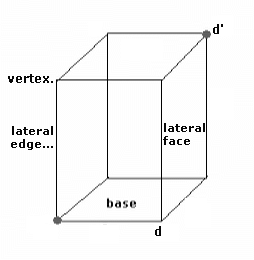### Prism

The Prism is a three-dimensional solid figure formed by two parallel polygons and congruent called bases. The number of sides of one of the bases gives the name of a prism, such as triangular, quadrangular, pentagonal and octagonal for 3, 4, 5 and 8 sides,respectively.
Isaac Newton, English physicist and mathematician, long time ago, used a triangular glass prism to show the decomposition of light, concluding, that, the white light is a mixture of seven colors: red, orange, yellow, green, blue, indigo and violet.
Prism elements:
• Face: parallelogram with four equal sides;
• Base: a polygonal figure;
• Total surface: sum of the all face areas;
• Lateral surface sum of the lateral face areas;
.   • Height: distance between the plane of a base and the othe. ;
• Face diagonal: the segments line, joining two opposite corners of the same face.
When the base of a prism is aligned directly above the two other, the prism is right. On the contrary is oblique.
If the base of a prism is a regular polygon, the prism is regular. On the contrary, the prism is irregular.
How to calculate the elements of a Prism.Was this information helpful? First name: Yes No Not sure